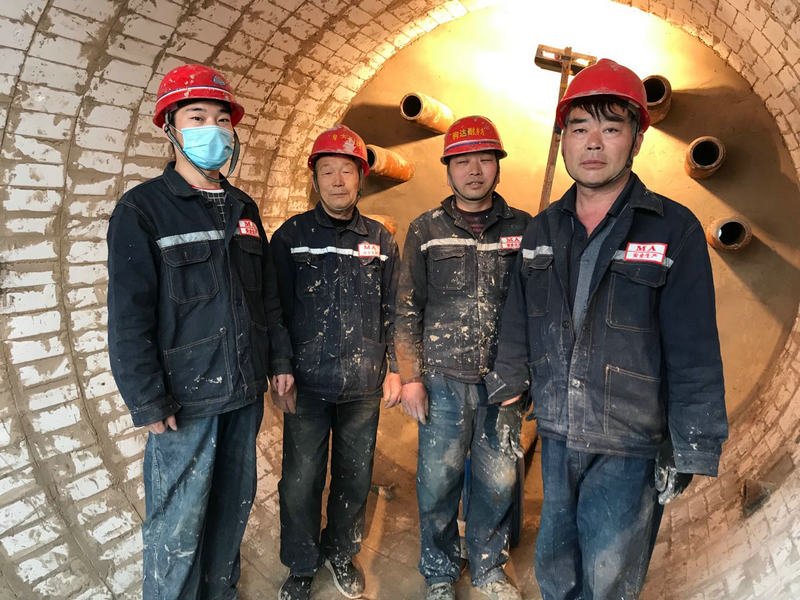# 影响制硫炉性能优劣 的最直接因素之一是炉内衬耐火材料

H2S+1/202=H20+1/2S2+Q

CH4+202=CO2+2H20+Q

4NH3+302=2N2+H20+Q

4NH3+502=4NO+H20+Q

2NH3=N2+3H2Q

H2S+CO2=COS+HE0Q

H2S+1/2C02=1/2CS2+H20Q

H2S+CO2=CO+S+H2OQ